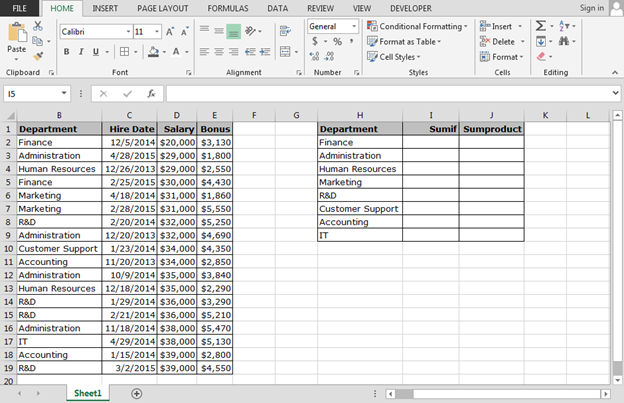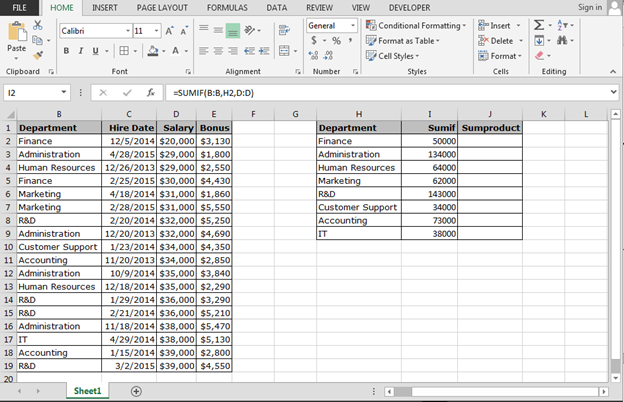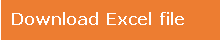# Using multiple criteria in SUMIF Function

In this article, we will learn how to use the multiple criteria in Sumif function.

Problem is: - Summing the total from cells in one column that meets the criteria based on a range of dates in another column.

This problem we can resolve through 2 Excel formulas:-

1) SUMIF function: -This function is used to sum up the cells on the basis of multiple criteria.

Syntax of SUMIF:-=SUMIF(range, criteria,[sum_range])

 Syntax =SUMIF(range, criteria,[sum_range]) Range Data range from which we want to retrieve the sum Criteria For which we want to calculate the sum from the data Sum_Range The range of column from which we want calculate the sum

1) SUMPRODUCT function: - This function is used to sum up the products for the corresponding ranges and array.

Syntax of SUMPRODUCT:-=SUMPRODUCT (array1, array2,array3,….)

Let’s take an example and understand how we can use SUMIF and SUMPRODUCT functions to resolve the problem.

We have HR data in which we have salary details of every employee, department wise. Now, we want to retrieve the total salary amount department wise.How to use SUMIF function for summing up the values on the basis of multiple criteria?

• Enter the formula in cell I2.
• =SUMIF(B:B,H2,D:D), and press Enter.
• Copy the same formula in the range.

Formula Explanation:-

1) B:B is the department range for which we want to pick the sum of salary.

2) H2 is the criteria for which formula will calculate the sum.

3) D:D is the sum range in the data.How to use SUMPRODUCT function for summing up the values on the basis of multiple criteria?

• Enter the formula in cell I2.
• =SUMPRODUCT((B2:B19=H2)*D2:D19), and press Enter.
• Copy the same formula in the range.

Formula Explanation:-

1) B2:B19=H2:- This syntax will help to match the criteria from the data.

2) D2:D19:- This syntax will retrieve the sum on the basis of first syntax.## Users are saying about us...

1.Do you want to read about the syntax and usage of an Excel 2013 or VBA function in your native language and practice with a demo workbook ?

The facts:
There are over 600 Excel & VBA functions in Office 2013.
Excel functions have been translated in 16 languages.
Microsoft offers over 20,000+ function help webpages in 50+ languages.

How to navigate fast among so many help pages ?

2.